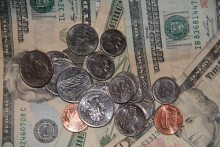# How Many Cents Equal One Dollar?Although the word 'dollar' can be traced back in its usage to mainland Europe as far back as the fifteen hundreds, being derived from currencies such as the Joachimstal of Bohemia, I am going to assume that the question is specifically about the American dollar, which has taken its place as not only the most prominent dollar, but also the most prominent currency in general from the early twentieth century. The very first United States dollars were issued in coin form in 1792 by the United States Mint, and today it is the currency of dozens of countries worldwide, from the Eastern Caribbean territories  to New Zealand. However, the country of origin makes no different to the number of cents in each dollar, since the ratio is always a constant. That is, there is always exactly one hundred cents in a single dollar. This consistency is a necessary condition of the cents' existence because if you translate it from the Latin word it is derived from - 'centum' - it means one hundred. Thus, it has become the  monetary unit for one hundredth of the basic monetary unit. To put it another way, when you talk about a cent, you are by definition talking about one hundredth of a dollar.
thanked the writer.
100 cents equal to one dollar
thanked the writer.
100 cents are equal to a dollar
thanked the writer.
Cent is a monetary unit in currencies. There are different currencies which have the cent as their smallest unit when measuring monetary value and in these currencies; there is a coin for a cent.

The two currencies which use cent as a unit are the US dollar and the Canadian dollar. A cent is 1/100 of a dollar which means that hundred cents make one dollar. Cent is represented by ¢
thanked the writer.
Two 50 cents equals one dollar. 50 cents is a good rapper.
thanked the writer.
You, I cent is equal to 1/100th Dollar or 100cents is equal to 1 Dollar.
thanked the writer.
100 cents for a dollar in Canada
thanked the writer.
May be 100 some times, but I am not that dam sure!!!
thanked the writer.
100 cents are equal to 1 dollar
thanked the writer.
100 cents equals 1 dollar
thanked the writer.
One dollar is 100 cents.
thanked the writer.
100 cents are equal to \$1.00
thanked the writer.
100 cents for a dollar in Canada^^
thanked the writer.
100 cents= 1 \$
thanked the writer.
100 cents = a dollar.
thanked the writer.
I don't know if this helps, but 100 pennies equal one dollar, 20 nickles equal one dollar, 10 dimes equal one dollar, and 4 quarters equal one dollar.
thanked the writer.
The symbol '\$' represents one dollar. It is official currency in many countries and regions. United States Dollar is widely used currency. One dollar amounts to hundred cents. Thus, one cent is the smallest unit of currency. This conversion is fixed world over. Thus, it is one / hundredth part of a dollar.

A cent literally means hundred and is taken from the Latin word centum. The word centum also means hundred. A cent is represented by 'c' or '¢'. The later sign is used by United States and Canada and the former by Australia, New Zealand and countries of Europe. Thus, only hundred cents make any dollar all over the world. Hundred cents make up one Arubian florin, Australian dollar, Bahamian dollar, East Carribean dollar, Fijian dollar, Liberian dollar or Singapore dollar.
thanked the writer.
100 cents equal 1 U.S dollar
thanked the writer.
100 cents equal 1 dollar
thanked the writer.
I feel so illiterate googling this
thanked the writer.
100 cents/pennies = \$1.00.
thanked the writer.
If you have a bunch of 10cents and 5 cents stamps, and you know there are 20 stamps and their total value is \$1.50, how many of each do you have?
thanked the writer.
I'm confused with your question. But I think the answer is 100 cents.
thanked the writer.
You could use 100 penny's 10 dimes 20 nickles 4 quarters
thanked the writer.
100 cents are there in 1\$
thanked the writer.
There are 100 cents in a dollar
thanked the writer.